# Beyond Stocks: The Surprising Volatility Returns of Oil and Gold

I’ve previously discussed the Volatility Risk Premium (VRP) and how it differs from the Equity Risk Premium (ERP).

Probably the most interesting difference, from the perspective of the trader, is that the VRP may be somewhat amenable to timing – more than the ERP at any rate.

In this article, I’ll use some of the excellent data from ORATS to explore the VRP.

We’ll start by using the SPY ETF to explore the VRP of the S&P500. This will also serve as an introduction to how we might actually do the analysis – there exists some awkwardness around aligning implied and realised volatilities for comparison, as you’ll see.

We’ll then move on to an analysis of the VRP across different sectors.

## The data

ORATS provide some fantastic products:

• Data API: Historical options data (bid/ask, volumes, open interest, greeks) across all strikes and expiries, and core research data (proprietary data for options research).
• Historical data via FTP
• Trading tools: stock and options scanners, options backtester, broker integration (Tradier and TD Ameritrade currently, Interactive Brokers coming), earnings dashboards and more.

In this article, we’ll use implied and realised volatility calculations from the core research data set.

ORATS have generously offered a discount on their products to readers of Robot Wealth. You can get up to 66% off using this link.

## Implied and realised volatilty: the VRP ingredients

Before we get to the good stuff, some definitions:

• Implied volatilty is a forward-looking estimate of the market’s expectations of volatility. It is derived (or implied) from the price of a stock’s options.
• Realised volatility is a measure of what actually happend – how much volatility was realised.
• The difference between implied and realised volatility is the VRP.

The VRP is positive, most of the time. Sometimes it is very negative.

Being short implied volatility essentially means being short volatility at the current market price. And since the VRP is positive most of time (that is, volatility tends to trade at a premium), being short volatility is therefore a good bet. Most of the time. Until it isn’t.

This chart from the previous article illustrates the point. It shows a constantly compounded short position in VIXY (the long volatility ETF) in the absence of short borrow costs and trading fees.It’s a case of stairs up, free-fall down.

### Implied and realised volatility of SPY

In this article, we’ll use sctor ETFs as proxies for sector implied and realised volatilities (IV and RV) and the VRP.

The IV, RV, and VRP of these ETFs are a decent proxy for the IV, RV, and VRP of their relevant sector or index because:

• They’re designed to track their index closely
• They’re generally quite liquid, which implies a liquid options market and more reliable IV and RV calculations.

However, there are a few nuances to consider:

• All ETFs will have some tracking error, meaning its returns could deviate slightly from the sector or index.
• Sector ETFs pay dividends, but the index itself is price return only. This can introduce small discrepancies when calculating realized volatilities.
• The mechanics of ETF trading and the role of authorized participants in creating and redeeming ETF shares can introduce idiosyncrasies that don’t exist for the index itself.

These are all likely to be small effects so long as we stick with liquid sector ETFs.

First, I’ll read in my ORATS API key from my .Renviron file:

# get orats key environment variable
source("../../.Rprofile")
ORATS_KEY <- Sys.getenv("ORATS_KEY")

Next I’ll load the libraries I’ll use and set some charting options:

library(tidyverse)
library(glue)
library(httr2)

# Set chart options
options(repr.plot.width = 14, repr.plot.height=7)
theme_set(theme_bw())
theme_update(text = element_text(size = 20))

Now I’ll build the endpoint I need to pull Core Research data for SPY, do the request, and have a look at the data that’s returned:

# build endpoint
this_ticker <- "SPY"
CORE_URL_TICKERS <-  glue('https://api.orats.io/datav2/hist/cores.csv?token={ORATS_KEY}&ticker={this_ticker}')
# get SPY core data
spy <- request(CORE_URL_TICKERS) %>%
req_perform() %>%
resp_body_raw() %>%

head(spy)
Rows: 4223 Columns: 340
── Column specification ────────────────────────────────────────────────────────
Delimiter: ","
chr    (3): ticker, sector, bestEtf
dbl  (318): assetType, priorCls, pxAtmIv, mktCap, cVolu, cOi, pVolu, pOi, or...
lgl   (14): etfIncl, sectorName, ernDate1, ernDate2, ernDate3, ernDate4, ern...
dttm   (1): updatedAt
date   (4): tradeDate, divDate, nextErn, lastErn

ℹ Use spec() to retrieve the full column specification for this data.
ℹ Specify the column types or set show_col_types = FALSE to quiet this message.

A tibble: 6 × 340
<chr><date><dbl><dbl><dbl><dbl><dbl><dbl><dbl><dbl><dbl><dbl><dbl><dbl><dbl><dbl><dbl><dbl><dbl><dttm>
SPY2007-01-037141.62141.370 83675130344511064120521061.142500.9604171.144971.059051.149181.142500.9604171.1449702007-01-03 20:50:00
SPY2007-01-047141.37141.670 65015134275722875221211481.265460.9730661.185141.088911.167591.265460.9730661.1851402007-01-04 20:50:00
SPY2007-01-057141.67140.540 63374136385112948021973591.000001.0294001.093011.029401.149181.000001.0294001.0930102007-01-05 20:50:00
SPY2007-01-087140.54141.190 647771383531 9469822037431.029401.0159301.116101.000001.169541.029401.0159301.1161002007-01-08 20:50:00
SPY2007-01-097141.19141.070122671140748911467522311201.237560.9160641.139221.000001.088911.237560.9160641.1392202007-01-09 20:50:00
SPY2007-01-107141.07141.540 64254147407315491122271991.109030.9550211.149181.000001.179691.109030.9550211.1491802007-01-10 20:50:00

We have quite a lot of data here. R tells us that we have more than 4,000 rows (>10 years of daily data) and 340 columns. Each column contains one of ORATS’ core research metrics. There is an absolute ton of stuff here. Read about this treasure trove of data in the ORATS docs.

R also informed us we have some problems with our data. Let’s take a look:

problems(spy) %>%
distinct(col, expected, actual)
A tibble: 2 × 3
colexpectedactual
<int><chr><chr>
96date in ISO86010000-00-00
98date in ISO86010000-00-00

Looks like we have two problematic columns – column 96 and 98. Let’s see what these are:

colnames(spy)[c(96, 98)]
1. ‘nextErn’
2. ‘lastErn’

The docs state that nextErn is the next earnings date and is available through another subscription. Likewise lastErn is last earnings date, also avilable through another subscription. I assume these were removed from the core data at some point, but the fields remain as placeholders.

We won’t use these fields, and there are no other issues with the data, so let’s carry on.

There are many ways to estimate IV and RV. Here, we’ll use ORATS’ iv30d metric for IV. This is a 30 calendar day interpolated implied volatility (interpolated because it uses more than one option expiry in its calculation). We’ll use orHv20d for RV. This is a 20 trading day historical intraday volatility.

Remembering that implied volatility is a forward-looking measure, calculating the VRP requires knowing how much volatility realised over the implied period, which we don’t know until after the fact.

Here’s a plot that makes this clearer. It shows time series of iv30d and orHv20d. I’ve labelled orHv20d as historical volatility for clarity:

spy_vrp <- spy %>%
mutate(
Implied = iv30d,
Historical = orHv20d
) %>%
na.omit()

example_end_date <- as_date("2020-06-30")

spy_vrp %>%
mutate(
Implied = case_when(tradeDate >= example_end_date ~ NA_real_, TRUE ~ Implied),
Historical = case_when(tradeDate >= example_end_date ~ NA_real_, TRUE ~ Historical)
) %>%
pivot_longer(c(-tradeDate, -ticker), names_to = "vol_type", values_to = "vol") %>%
ggplot(aes(x = tradeDate, y = vol, colour = vol_type)) +
geom_line() +
geom_vline(xintercept = example_end_date, colour = "red", linewidth = 2) +
geom_segment(
x = example_end_date, xend = example_end_date+days(60), y = 30, yend = 30,
colour = "black", arrow = arrow(type = "closed"), show.legend = FALSE
) +
geom_text(x = example_end_date+days(75), y = 30, size = 6, colour = "gray30", label = "Implied Vol. forecasts this", vjust = -1, hjust = 0.5, show.legend = FALSE) +
geom_segment(
x = example_end_date, xend = example_end_date-days(60), y = 20, yend = 20,
colour = "black", arrow = arrow(type = "closed"), show.legend = FALSE
) +
geom_text(x = example_end_date-days(75), y = 20, size = 6, colour = "gray30", label = "Historical Vol. estimates this", vjust = 2, hjust = 0.5, show.legend = FALSE) +
labs(
title = "Implied and historical realised SPY volatility",
x = "Date",
y = "Volatility",
colour = "Vol.Type"
)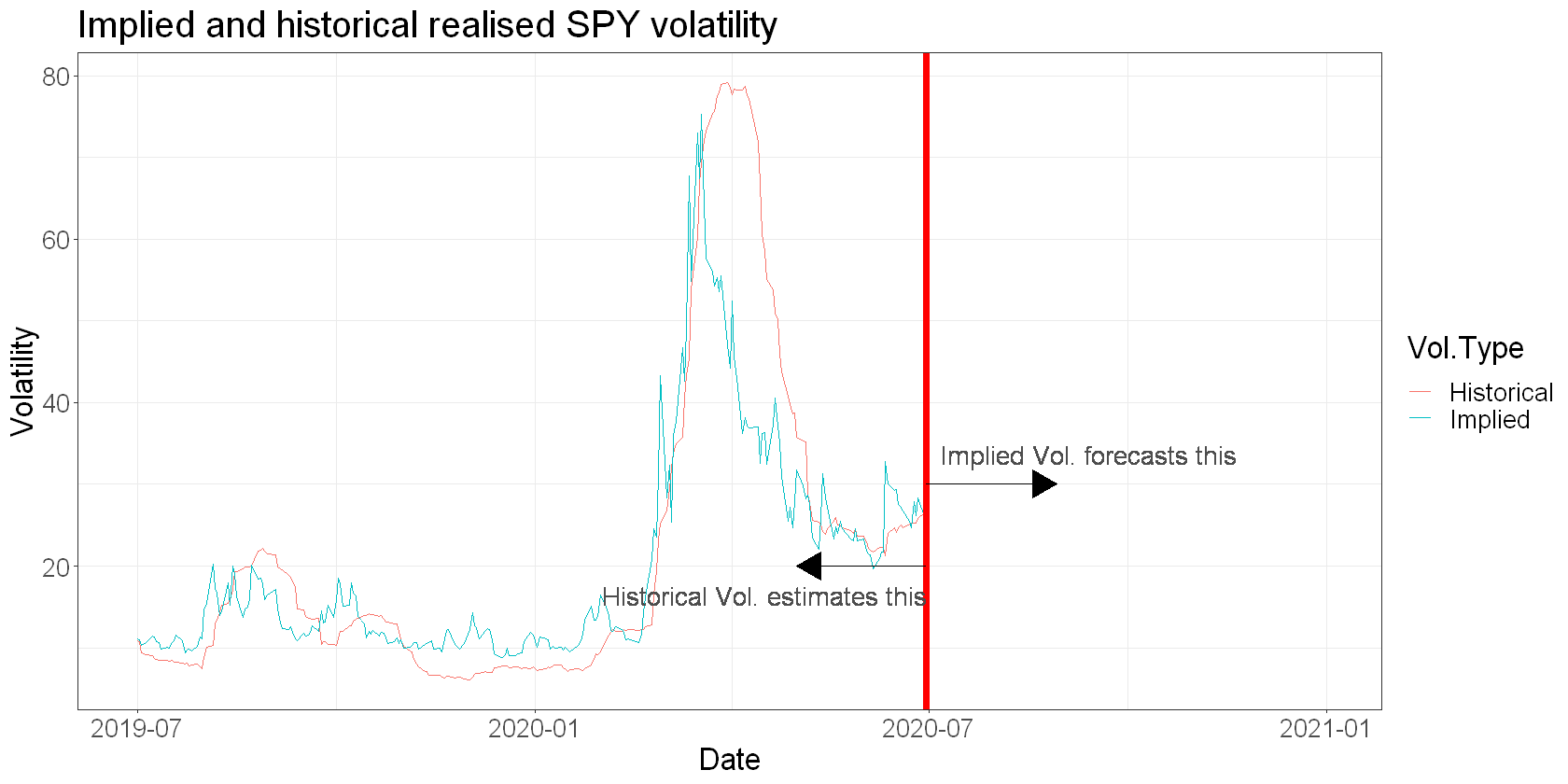You can see that early in 2020, forward-looking implied volatility shot up above the estimate of historical volatility. It then came down fairly quickly as the options market repriced the forward risk following the initial covid panic.

And since the historical volatility estimate consists of a moving window of data, you can see that it remained elevated for some time even as implied volatility came down, since by definition it takes time for some of those high-volatility days to drop out of the estimation window.

I think this illustrates nicely some of the practical differences between implied and realised volatility estimates:

• Implied is by definition forward looking; realised is backwards looking.
• Implied can change relatively quickly since it reflects current options prices.
• Realised can only change as a function of its estimation window, which will by definition reflect slower changes than we see in implied.

These differences spill over into our estimation of the VRP. Note that like realised volatility, the VRP is something we can’t measure directly, but can only estimate. Any estimate of the VRP will by definition be subject to how we estimate our realised volatility and calculate our implied volatility.

The first thing we need to consider is aligning our realised volatility estimate with our implied volatilty.

Calculating the VRP requires estimating how much volatility realised over the period of our implied volatility measure. That means shifting one of our series – we could shift our historical volatility estimate forward, or we could shift our implied volatility backwards.

But complicating matters is the fact that implied volatility is normally measured over some number of calendar days, reflecting time to options expiration, while historical volatility is estimated over some number of trading days.

This is why I chose a 30-day implied volatility and a 20-day realised volatility. 30 calendar days is approximatley equal to $5\mathrm{/}7\ast 30=215/7 * 30 = 21$ trading days, without considering holidays.

While the periods aren’t exactly the same, at least they’re close enough to be comparable.

Aligning the volatility is a bit tricky, but an approach that gets us most of the way there is to insert rows for the missing calendar dates, and then fill the resulting NA volatility values. This way, you can directly shift the implied volatility by 30 calendar days without having to adjust for trading days. This won’t align the periods perfectly, but gets us close enough to do some analysis in the aggregate:

spy_vrp <- spy %>%
mutate(
Implied = iv30d,
# I'm also changing the name of Historical volatility to Realised to better reflect what we're doing here
Realised = orHv20d
) %>%
na.omit()

# first, create a full sequence of dates from the min to the max date
all_dates <- data.frame(date = seq(min(spy_vrp$tradeDate), max(spy_vrp$tradeDate), by="1 day"))

# then, left join the spy data on the full sequence of dates
spy_vrp <- all_dates %>%

# spy_vrp now contains rows for all calendar dates with NA for missing trading days
# next, we shift IV by 30 calendar days and remove any left over NA values:
spy_vrp <- spy_vrp %>%
mutate(Implied = lag(Implied, 30)) %>%
na.omit()

Let’s now plot our aligned implied and realised volatility over the full sample:

# time series plots
spy_vrp %>%
pivot_longer(c(-date, -ticker), names_to = "vol_type", values_to = "vol") %>%
ggplot(aes(x = date, y = vol, colour = vol_type)) +
geom_line() +
labs(title = "Implied and realised 20-day SPY volatility")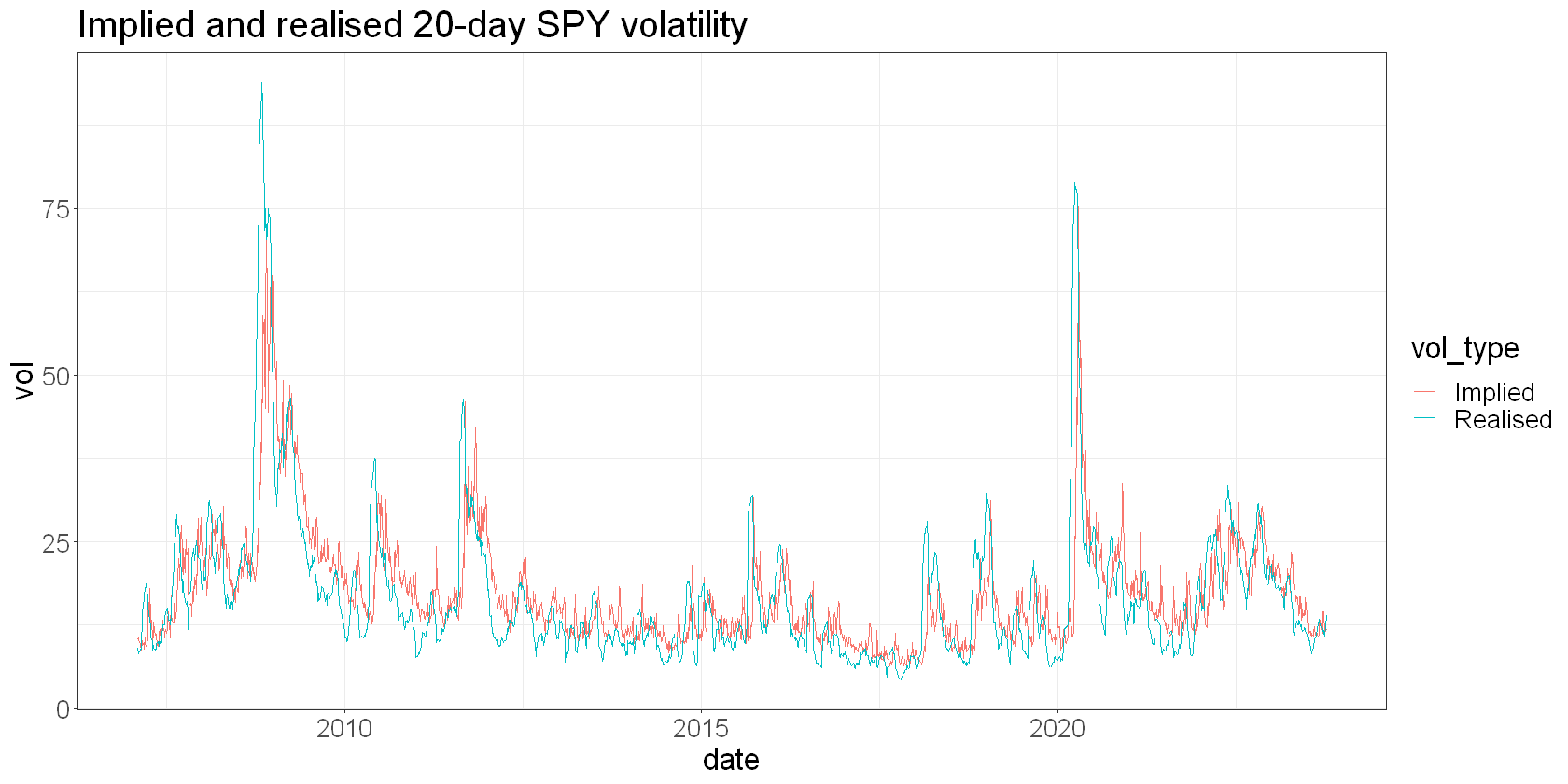Eyeballing this plot suggests that implied volatility usually exceeds realised volatility. But sometimes things go a bit haywire and realised spiked above implied.

This is important, because if implied is persistently greater than realised, then selling volatility should realise the VRP and generate a profit. But anyone that’s sold options will recognise those spikes in realised volatility as times when they got absolutely whacked.

If we can predict when implied is more likely to be greater than realised, then it stands to reason that we could do better selling volatility.

If we remove overalapping data (that is, plot only a single point from any 20 trading day window) and make a scatter plot of implied vs realised volatility, we can get a sense of how the VRP behaves for SPY.

In the plot below:

• Points above the line represent a positive VRP (short vol made money)
• Points below the line represent a negative VRP (short vol lost money)
• The orange line represents where implied and realised volatilities are equal
• The further a point is from the line, the greater the volatility premium or discount
spy_vrp %>%
filter(row_number() %% 20 == 0) %>%
ggplot(aes(x = Realised, y = Implied)) +
geom_point() +
geom_abline(intercept = 0, slope = 1, colour = "darkorange2", linewidth = 2) +
labs(title = "Volatility Premia and Discounts, SPY")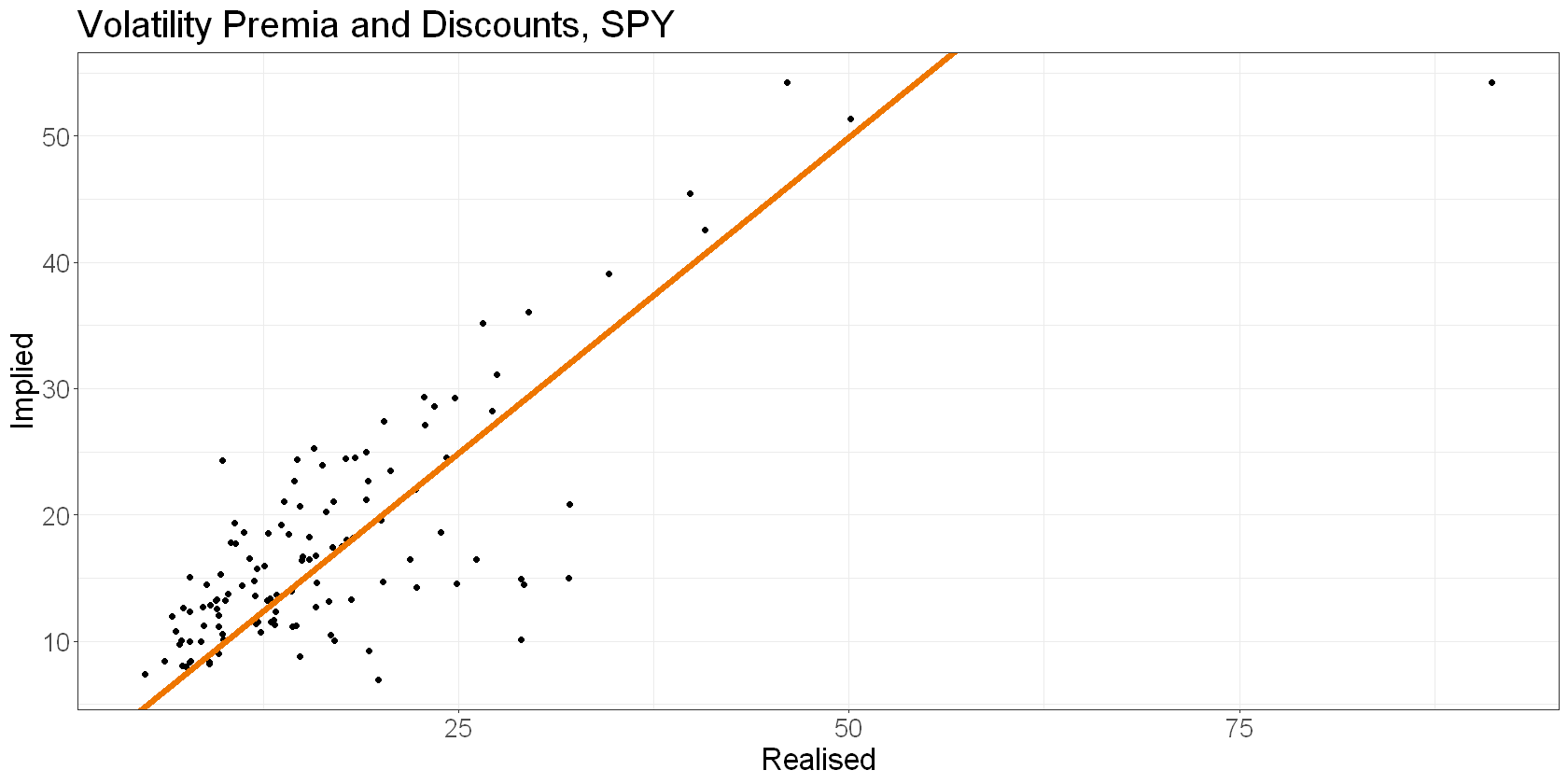You can see that implied is greater than realised most of the time (more points above the orange line).

But also notice that the points above the line tend to be clustered closer to the line. On the other hand, points below the line can often be far away.

That corresponds to the “picking up pennies in front of a steam-roller” feeling that people describe when they sell volatility. They make a little money most of the time, and ocassionally get run over.

The goal of timing the VRP would be to avoid those points below the orange line. Or even flip and get long volatility.

Next let’s calculate the VRP and plot it as a time series:

spy_vrp <- spy_vrp %>%
mutate(VRP = Implied - Realised)

spy_vrp %>%
ggplot(aes(x = date, y = VRP)) +
geom_line() +
geom_hline(yintercept = 0, colour = "darkorange2", size = 1.5) +
labs(title = "SPY VRP")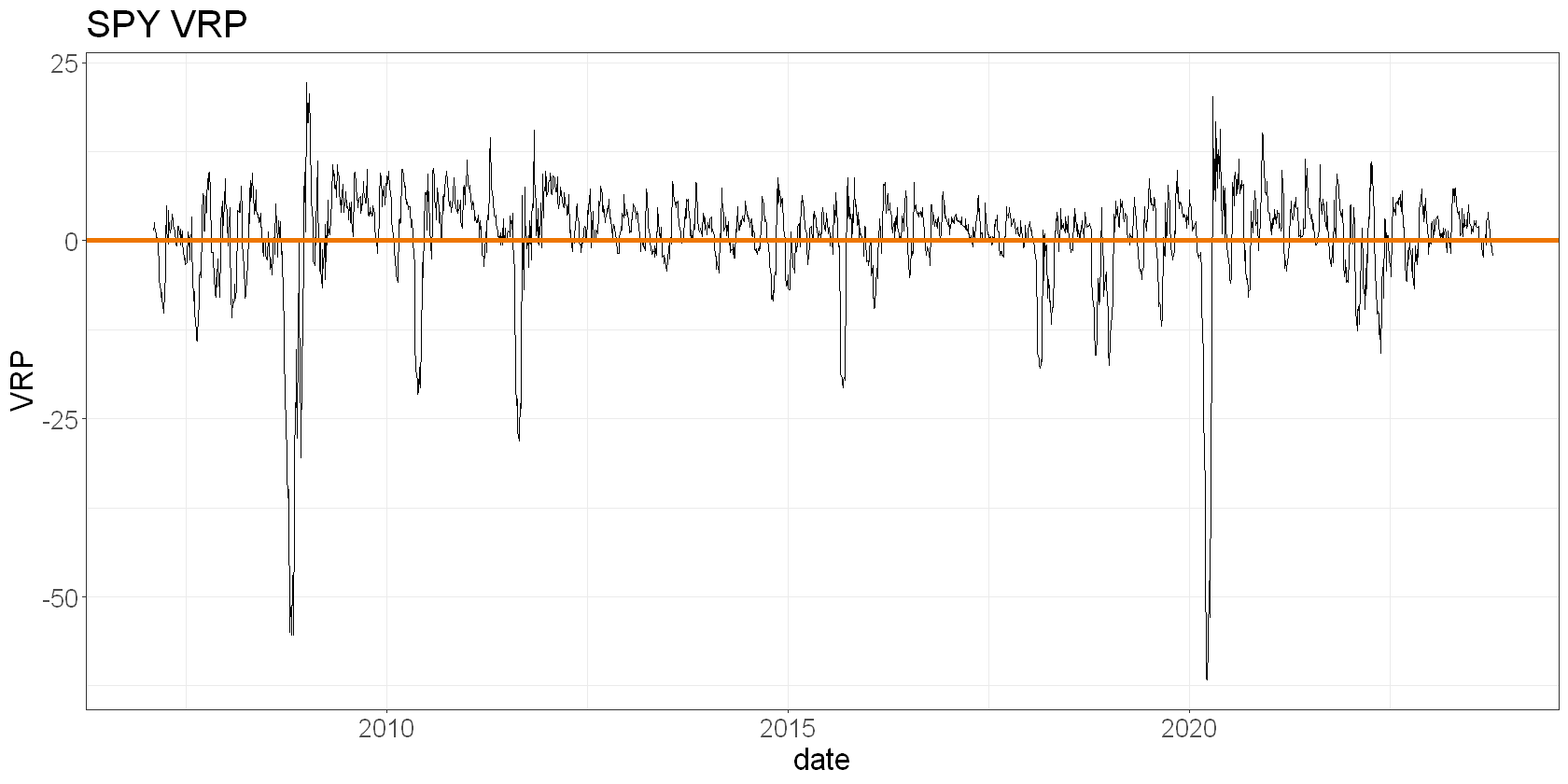We see the VRP behaviour even more clearly in the time series: most of the time, we see a small, positive VRP, and occassionally we see a very negative one. Interestingly, we often see the highest positive VRP immediately after a very negative period. Does that represent the options market remaining in panic mode slightly too long?

Next I’d like to know a little about the returns to being short SPY volatility. Specifically, I want to know what the average return was, as well as the distribution of those returns – in particular, their spread and skew.

Volatility itself isn’t directly interpretable as a return, but one interpretation of the VRP is the compensation volatility sellers demand for the uncertainty of future volatility. Therefore, we could think about the VRP as the excess return for being short volatility, and get a hacky estimate by calculating the VRP as a percentage of implied volatility, which we could interpret as the size of a premium or discount the market is delivering relative to its expectations.

Admittedly this approach is very hand-wavy. But I think it’s OK as a relative measure – that is, for making comparisons. I wouldn’t consider it representative of actual returns (shortly I’ll use it to compare the VRP across sector ETFs).

spy_vrp <- spy_vrp %>%
mutate(
VRP_return_pct = VRP/Implied
)

What’s the mean “return” to the VRP?

mean_spy_vrp_return <- spy_vrp %>%
summarise(
mean_return = mean(VRP_return_pct)/100,
cagr = (1 + mean_return)^252 - 1
)

mean_spy_vrp_return
A data.frame: 1 × 2
mean_returncagr
<dbl><dbl>
0.00015831220.04069787

That’s a mean daily “return” of 0.016% and a “CAGR” of 4.1%.

I used quotation marks to remind us that these values don’t represent actual returns, but that they’re useful in a relative sense.

As well as the mean “return”, I’m also interested in the spread of returns:

spy_vrp %>%
ggplot(aes(x = VRP_return_pct)) +
geom_histogram(bins = 50) +
geom_vline(xintercept = pull(mean_spy_vrp_return, mean_return), linetype = "dashed", colour = "red", linewidth = 1.5) +
labs(
title = "Histogram of \"returns\" to SPY VRP",
subtitle = "Dashed line shows mean return"
)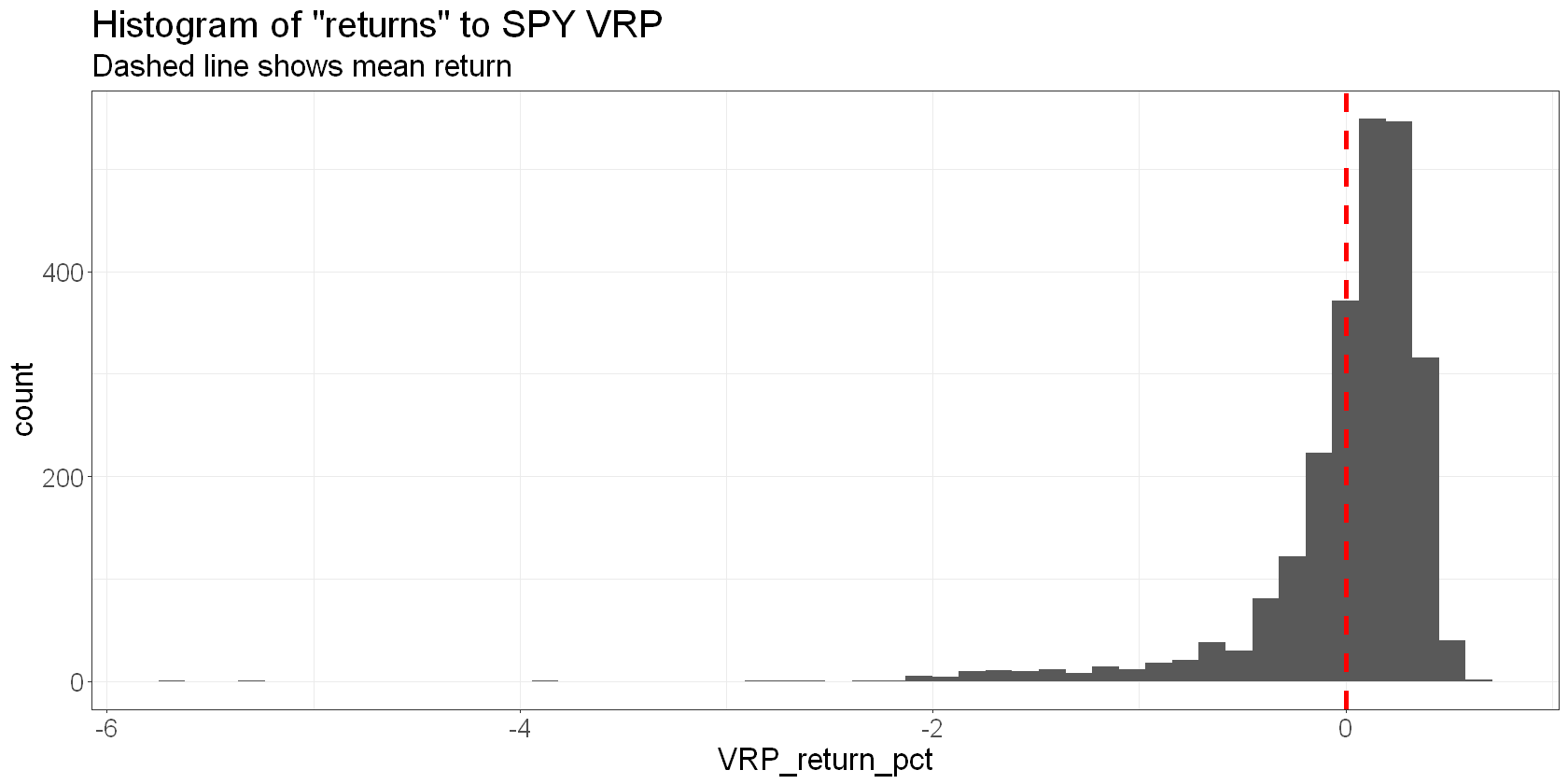This in my opinion is the really interesting chart. We see a small positive mean return, a lot of small positive returns and a smaller number of large negative returns. We see in some cases that realised volatility exceeded 5x the market’s expectations!

Let’s visualise the aggregation of these “returns” through time. Again, this doesn’t represent actual returns and it certainly isn’t a backtest, but it will be useful in a relative sense:

spy_vrp <- spy_vrp %>%
mutate(
VRP_return_log = log(VRP_return_pct/100 + 1),
cum_VRP_return = cumsum(VRP_return_log)
)

spy_vrp %>%
ggplot(aes(x = date, y = cum_VRP_return)) +
geom_line() +
labs(
title = "Cumaulative \"returns\" to SPY VRP",
y = "Cumulative \"return\""
)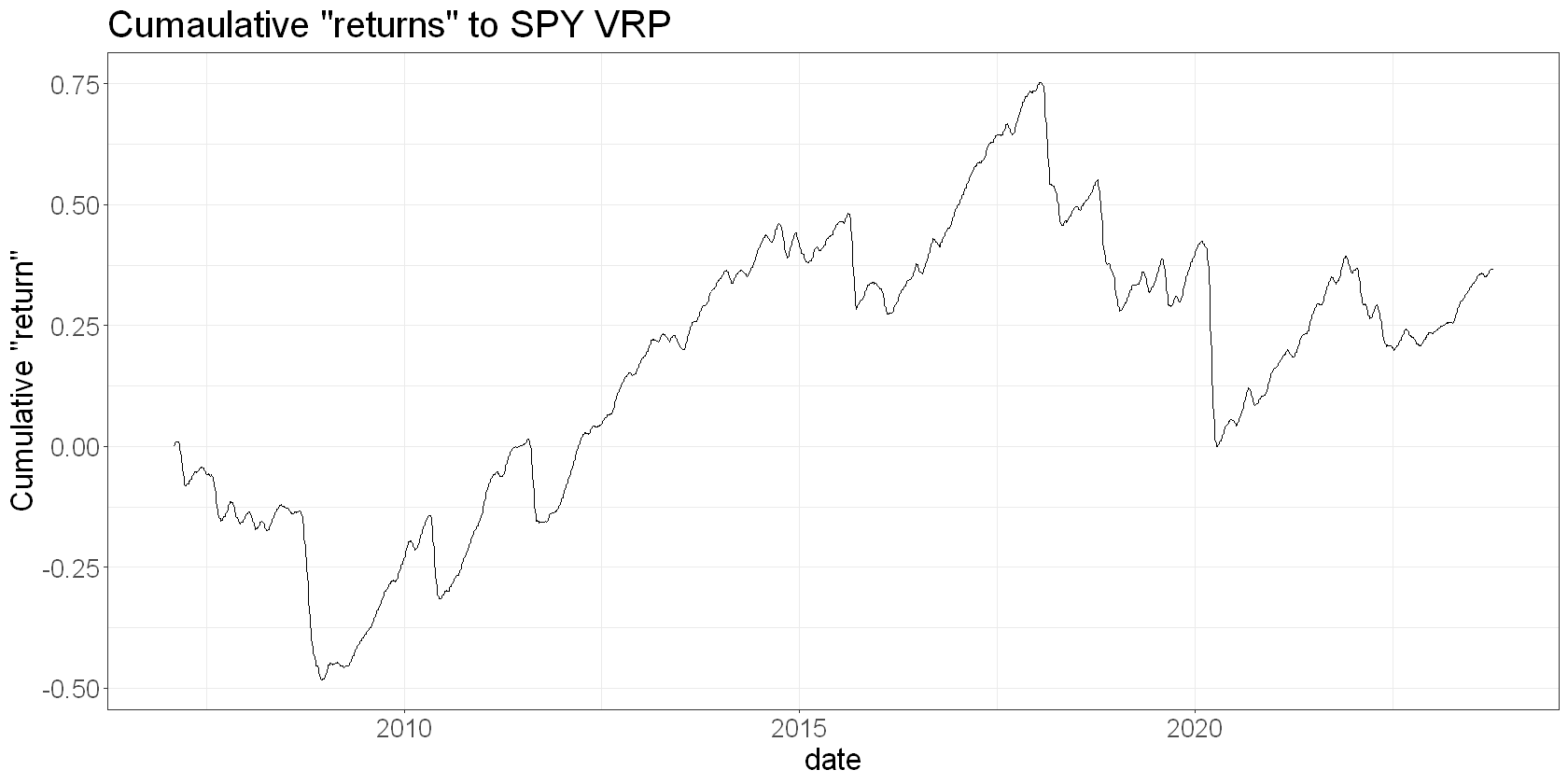Next, I’d like to compare this to other sector ETFs in order to figure out if any sectors tend to show a persistently higher VRP than other sectors (at least historically).

We’ll look at the mean as well as the distribution of “returns” to the VRP for various sector ETFs. I suspect we will find a trade off where the higher the average VRP return, the more extreme the spread and skew of that return.

Let’s find out.

# functions for wrangling core research data into IV, RV and VRP

get_core_research <- function(ticker) {
request(glue('https://api.orats.io/datav2/hist/cores.csv?token={ORATS_KEY}&ticker={ticker}')) %>%
req_perform() %>%
resp_body_raw() %>%
}

make_vrp_df <- function(ticker) {
vrp = get_core_research(ticker) %>%
mutate(
Implied = iv30d,
Realised = orHv20d
) %>%
na.omit()

# create a full sequence of dates from the min to the max date
all_dates = data.frame(date = seq(min(vrp$tradeDate), max(vrp$tradeDate), by="1 day"))

# then, left join the spy data on the full sequence of dates
vrp = all_dates %>%

# vrp now contains rows for all calendar dates with NA for missing trading days
# next, we shift IV by 30 calendar days and remove any left over NA values:
vrp = vrp %>%
mutate(Implied = lag(Implied, 30)) %>%
na.omit() %>%
mutate(
VRP = Implied - Realised,
VRP_return_pct = VRP/Implied,
# log return
VRP_return_log = log(VRP_return_pct/100 + 1),
# cumulative return
cum_VRP_return = cumsum(VRP_return_log)
)

vrp
}

# list of sectors
sectors <- c(energy = "XLE", financials = "XLF", utilities = "XLU", industrials = "XLI", technology = "XLK", gold = "GLD", oil = "USO")

# make vrp dataframe with all the sector ETFs
vrp <- sectors %>%
map_dfr(~purrr::quietly(make_vrp_df)(.x)\$result, .id = 'name') %>%
arrange(date, ticker)

head(vrp)
A data.frame: 6 × 9
namedatetickerImpliedRealisedVRPVRP_return_pctVRP_return_logcum_VRP_return
<chr><date><chr><dbl><dbl><dbl><dbl><dbl><dbl>
1energy 2007-02-02XLE27.7324.133.600.129823300.00129739100.0012973910
2financials 2007-02-02XLF12.2810.192.090.170195440.00170050770.0017005077
3industrials2007-02-02XLI13.2612.171.090.082202110.00082168340.0008216834
4technology 2007-02-02XLK14.4714.050.420.029025570.00029021360.0002902136
5utilities 2007-02-02XLU11.1410.250.890.079892280.00079860380.0007986038
6energy 2007-02-07XLE27.2123.393.820.140389560.00140291110.0027003021

Now that we’ve got our sector VRP data, let’s look at some aggregate results:

vrp %>%
group_by(name) %>%
summarise(
num_obs = n(),
mean_vrp_return = mean(VRP_return_pct/100),
sd_vrp_return = sd(VRP_return_pct/100)
)
A tibble: 7 × 4
<chr><int><dbl><dbl>
energy 2448-0.00007168020.003403557
financials 2448 0.00019703340.004401029
gold 2241 0.00145177390.002410457
industrials2448 0.00031557560.003566499
oil 2396 0.00109843790.002522938
technology 2448 0.00012371870.004133202
utilities 2448-0.00083805560.004336949

Interesting! By this measure, energy and utilities had negative average volatility risk premia!

Gold and oil had the highest mean VRP, a full order of magnitude greater than financials and technology. Surprisingly, their VRP also had a narrower distribution. Let’s confirm this with a density plot for each sector’s VRP “returns”:

# plot cumulative returns, spread, mean
vrp %>%
ggplot(aes(x = VRP_return_pct, fill = name)) +
geom_density() +
facet_wrap(~name) +
labs(title = "Distribution of VRP returns by sector")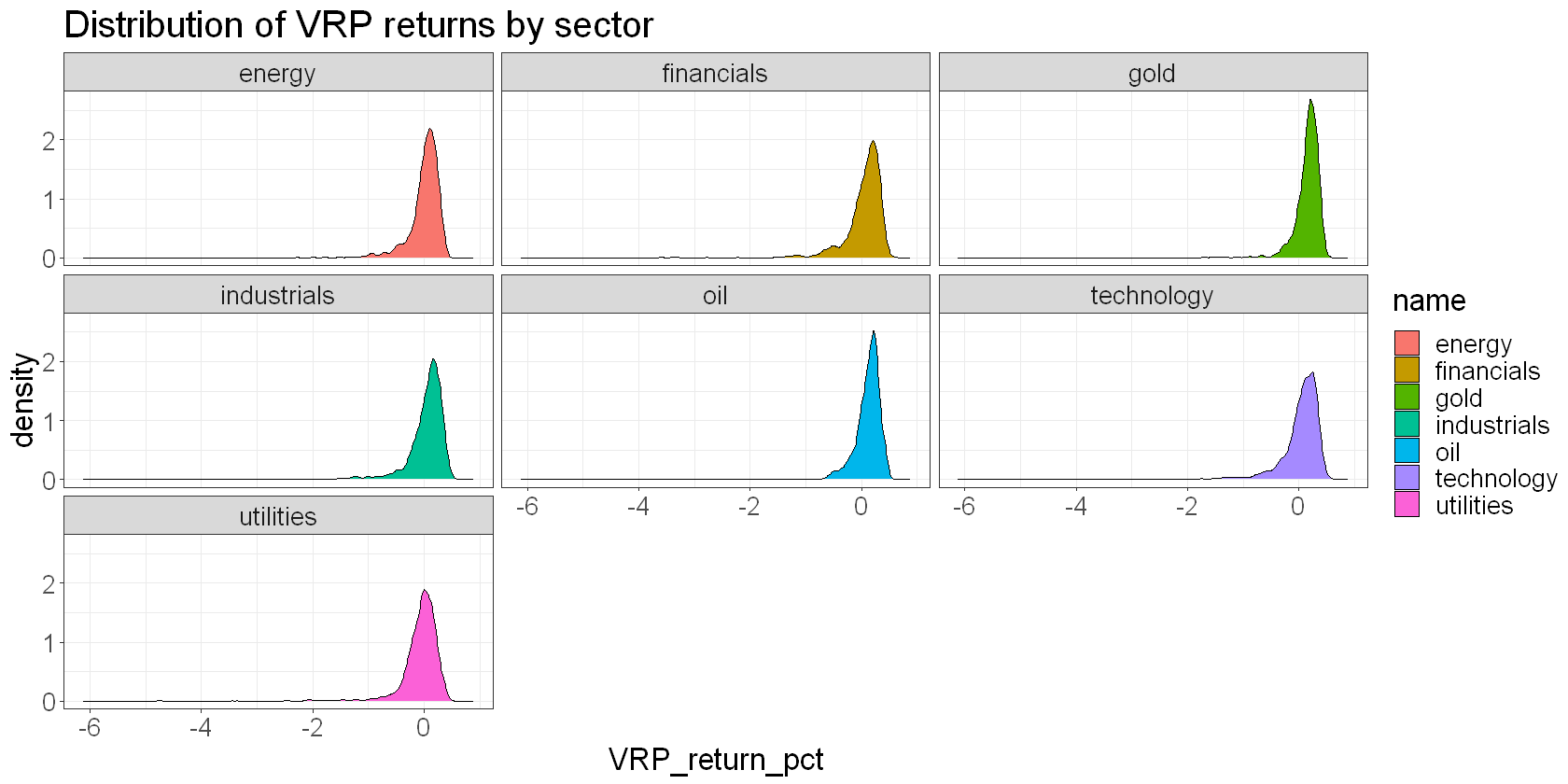Finally, a really effective way to compare sector VRP is plot the cumulative VRP “returns” and compare:

vrp %>%
ggplot(aes(x = date, y = cum_VRP_return, colour = name)) +
geom_line() +
labs(
title = "VRP \"returns\" by sector",
x = "Date",
y = "VRP \"returns\""
)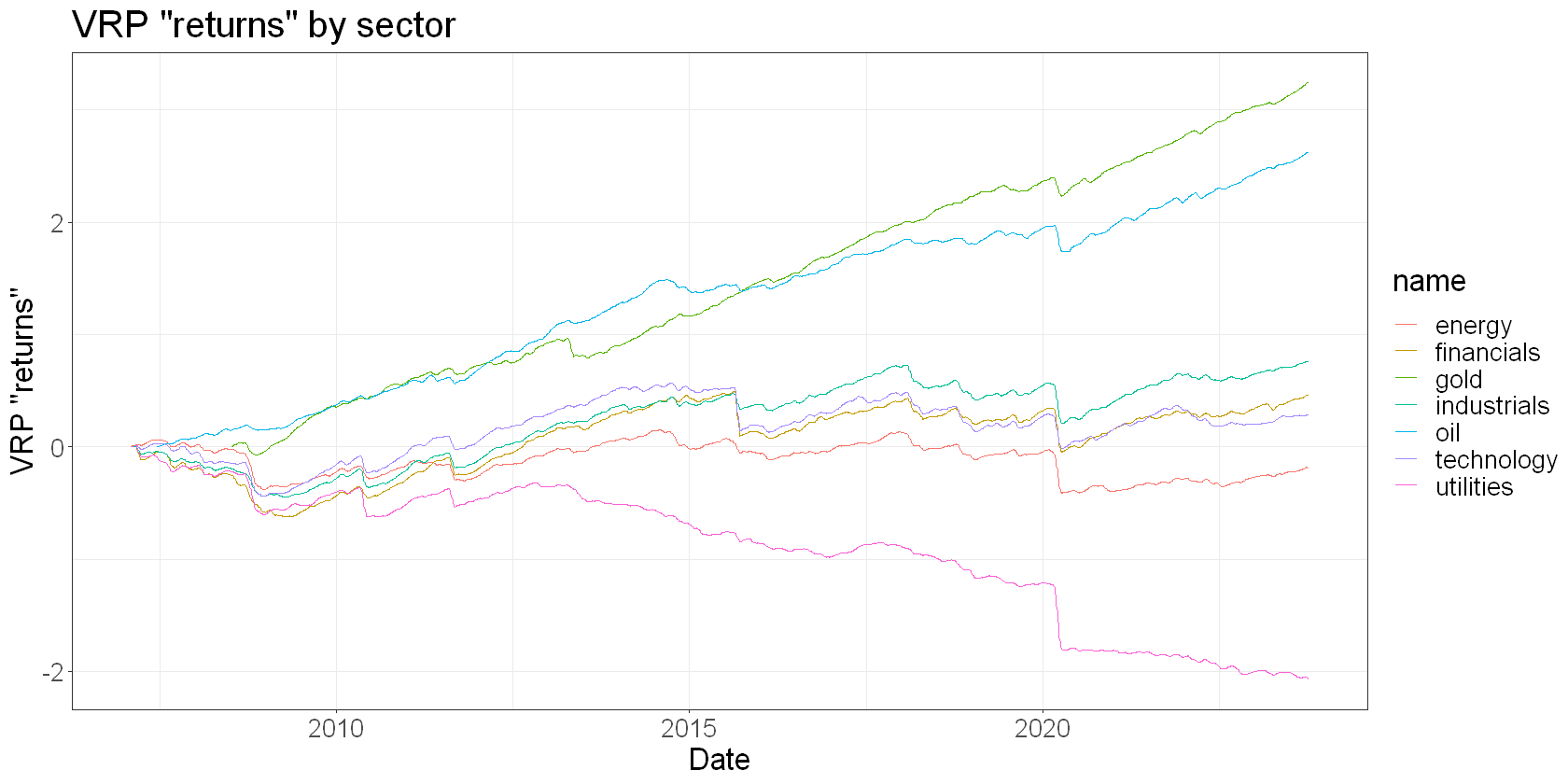The oil and gold ETFs have delivered much higher cumulative VRP “returns” than the other sectors (remembering that our “returns” are really only valid in a relative sense, ie for making comparisons).

I am somewhat surprised by these results.

On the one hand, it makes sense that a higher VRP is demanded for the volatile oil and gold sectors. These commodities tend to show a lot of volatility, and option writers therefore demand more premium.

On the other hand, I wouldn’t have intuited that this higher premium would actually compensate for that higher underlying volatility as well as it has. In particular, I expected to see a higher negative skew in these ETFs’ VRPs.

Of course, there’s a chance that the approximations and assumptions I’ve used in this analysis are the real reason the results are as they are. But I think I’ve seen enough here to warrant some deeper investigation.

## Summary

In this article we used two of the 340 fields in the ORATS core research data set to explore the VRP of the S&P500 and various sectors using ETFs as proxies.

We saw some of the practical issues around estimating the VRP, in particular how we might align forward looking implied volatility over some number of calendar days, with backwards looking realised volatility estimated over some number of trading days.

We discovered some things about the nature of the VRP:

• It’s usually positive and small
• Sometimes it goes negative
• When it goes negative, its magnitude tends to be much greater than when it’s positive

We made a proxy for VRP returns by expressing the VRP as a percentage of implied volatility – the amount it exceeds or falls beneath market expectations. We wouldn’t use this proxy to measure actual returns, but it is useful in a relative sense.

We found that the oil and gold ETFs have historically shown much higher VRP returns, and delivered them with less volatility, than other sectors.

## Two Announcements

### Discounted ORATS data

If you’d like to get your hands on the sensational ORATS data, you can get an incredibly generous 50-66% discount using this link. Many thanks to the team at ORATS for making this available to readers of Robot Wealth.

### Trade Like a Quant / Quant Like a Trader is back!!

Our hugely successful quant trading Bootcamp will open for enrolments on the 25th of October. The course is unique in that it teaches the fundamentals of running a quant trading operation as a part-time independent trader. You’ll learn:

• A mental model of the markets and its players
• The three mortal sins that you must avoid
• The things that need to be true in order to make money with a trading strategy
• How to structure a quant trading portfolio, starting with high-probability, uncompetitive edges and then layering alpha trades on top
• How to think about portfolio construction.

We’re very proud to focus on concepts you can use independently – how to think about the markets, find edges, and manage a portfolio. To use an old metaphor, to teach you to fish so that you may feed yourself forever. But we’ll also give you some fish, in the form of some things to trade, if you wish, so that you can hit the ground running with some stuff that we’re trading ourselves.